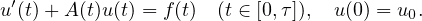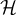Electron. J. Differential Equations, Vol. 2020 (2020), No. 124, pp. 1-24.

### Maximal regularity for non-autonomous Cauchy problems in weighted spaces Achache Mahdi, Tebbani Hossni

Abstract:
We consider the regularity for the non-autonomous Cauchy problemThe time dependent operator A(t) is associated with (time dependent) sesquilinear forms on a Hilbert space. We prove the maximal regularity result in temporally weighted L^2-spaces and other regularity properties for the solution of the problem under minimal regularity assumptions on the forms and the initial value u_0. Our results are motivated by boundary value problems.

Submitted October 9, 2019. Published December 20, 2020.
Math Subject Classifications: 35A23.
Key Words: Maximal regularity; non-autonomous evolution equation; weighted space.
DOI: 10.58997/ejde.2020.124

Show me the PDF file (415 KB), TEX file for this article.Achache Mahdi Department of Mathematics, Univ. Bordeaux Institut de Mathématiques (IMB). CNRS UMR 5251. 351 Cours de la Libération 33405 Talence, France email: Mahdi.Achache@math.ubordeaux.fr Tebbani Hossni Department of Mathematics Univ. Séetif -1-, Algeria email maths47@ymail.com